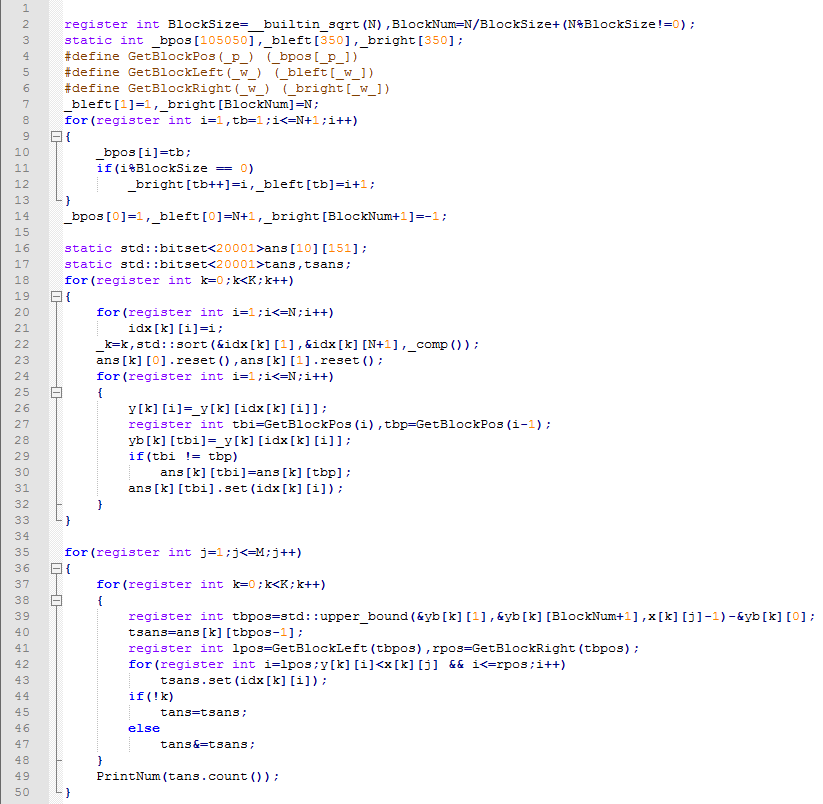[研学] 偏序问题的研究

• 高维偏序…

[ARC092D] Two Sequences

Time Limit: 3 sec / Memory Limit: 256 MB

• Problem Statement

You are given two integer sequences, each of length $$N$$: $$a_1, \cdots ,a_N$$ and $$b_1, \cdots ,b_N$$.

There are $$N^2$$ ways to choose two integers $$i$$ and $$j$$ such that $$1 \leq i,j \leq N$$. For each of these $$N^2$$ pairs, we will compute $$a_i+b_j$$ and write it on a sheet of paper. That is, we will write $$N^2$$ integers in total.

Compute the XOR of these $$N^2$$ integers.

• Input

$$n$$ 和两数组 $$a,b$$ 。

$$1 \leq … YZOJ P4637 [CSP-S 2019 五校联训 Round 2]由比滨结衣(sqrt) YZOJ P4637 [CSP-S 2019 五校联训 Round 2]由比滨结衣(sqrt) 时间限制：2000MS 内存限制：524288KB 难度：\(6.5$$

• 题目描述

1 l r x 将区间 $$[l,r]$$ 中的所有数变为 $$x$$。

2 l r x 查询区间 $$[l,r]$$ 中数字 $$x$$ 的出现次数。

• 题目描述

$$toggle i$$：切换第 $$i$$ 个路灯的状态。具体地说，若路灯原来亮起，则现在将熄灭；若路灯原来熄灭，则现在将亮起。

$$query a b$$：自动驾驶的士部门的负责人想知道，从 $$0$$ 时刻起到当前时刻，有多少个时刻满足：自动驾驶的士能够从站点 $$a$$ 出发到达站点 $$b$$ 。

• 输入格式

$$toggle i$$ $$(1 \leq i \leq n)$$：该时刻切换了第 $$i$$ 个路灯的状态。

$$query a b$$ $$(1 \leq a < b \leq n+1)$$：计算从 $$0$$ 时刻起到该时刻，共有多少个时刻满足的士能从站点 $$a$$ 出发到达站点 $$b$$ 。

…

YZOJ P2905 [PA2014]Druzyny

• 题目描述

OI组的神犇们决定把这题改造一下：

dick32165401：改成只有编号连续的的一段才可以分一组。

runzhe2000：判定可行解可能会被爆搜水过，最大化分的组数就不那么容易水过了。

E.Space：不仅要最大化组数，还要求出最大化组数的方案数。

ct：数据范围就出100万好了。

• 样例输出

Source: BZOJ 3711

YZOJ P3195 [NOI2017]游戏

• 题目描述

$$n$$ 场游戏的地图可以用一个小写字母组成的字符串描述。例如：$$S=\underline{\mathrm{xaabxcbc}}$$ 表示小 L 计划进行 $$8$$ 场游戏，其中第 $$1$$ 场和第 $$5$$ 场的地图类型是 $$x$$，适合所有赛车，第 $$2$$ 场和第 $$3$$ 场的地图是 $$a$$，不适合赛车 $$A$$，第 $$4$$ 场和第 $$7$$ 场的地图是 $$b$$，不适合赛车 $$B$$，第 $$6$$ 场和第 $$8$$ 场的地图是 $$c$$，不适合赛车 $$C$$ 。

• 输入格式

$$n,d,S$$ 的含义见题目描述，其中 $$S$$ 包含 $$n$$ 个字符，且其中恰好 $$d$$ 个为小写字母 $$x$$ 。